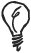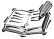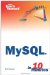# Data Types

When you create a new table, you must decide on the data types for each column. You already encountered some of the general column types, such as VARCHAR and INT, in Lesson 14. Now let's look at the most common data types in more detail.For a complete list of the data types you can use, refer to Appendix D, "MySQL Data Type Reference," which can be found on the book's website at www.samspublishing.com.

#### INTEGER

An integer is a whole number. The range of values that can be stored in an integer data type depends on the size of the integerMySQL has five different sizes:

• TINYINT can store numbers from 128 to 127, or 0 to 255 if unsigned.

• SMALLINT can store numbers from 32,768 to 32,767, or 0 to 65,535 if unsigned.

• MEDIUMINT can store numbers from 8,388,608 to 8,388,607, or 0 to 16,777,215 if unsigned.

• INTEGER or INT can store numbers from 2,147,483,648 to 2,147,483,647, or 0 to 4,294,967,295 if unsigned.

• BIGINT can store numbers from 9,223,372,036,854,775,808 to 9,223,372,036,854,775,807, or 0 to 18,446,744,073,709,551,615 if unsigned.## Unsigned Values

If an integer column is declared UNSIGNED, then it cannot store negative values. Doing so doubles the maximum values it can store.

In the sample database, quantity is defined as TINYINT UNSIGNEDit is expected that no single order will include more than 255 of the same product. The column is declared UNSIGNED because it is nonsense to allow a negative quantity.

#### DECIMAL

DECIMAL is an exact, fixed-point number. It is declared as DECIMAL(M,D), where M is the total number of digits and D is the number of digits after the decimal point.

In the sample database, we used DECIMAL for both the weight and price columns on the products table. Each was declared as DECIMAL(6,2), meaning that the largest value that could be stored in each is 9999.99six numbers in total, with two appearing after the decimal point.Sams Teach Yourself MySQL in 10 Minutes
ISBN: 0672328631
EAN: 2147483647
Year: 2006
Pages: 165
Authors: Chris Newman

Similar book on Amazon